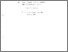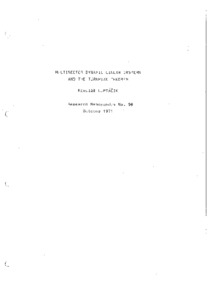# multisector dynamic linear systems and the turnpike theorem

Luptacik, Mikulas (October 1971) multisector dynamic linear systems and the turnpike theorem. Closed Series > Forschungsberichte / Research Memoranda 59Preview
Text
fo59.pdf

Download (7MB) | Preview

## Abstract or Table of Contents

abstract: the subject of this study is an analysis of two of the basic linear systems; leontief's system and von neumann's model. the properties of the dual prices in a turnpike example are examined. the chapters in this study are organized as follows: chapter i furnishes the mathematical background for the subsequent chapters and requires the basic knowledge in linear algebra. the mathematical content of chapter i is (1) the theory of nonnegative matrices and the conditions for the existence of nonnegative solution to systems of linear equations or inequalities of economic significance (the system in leontief's input-output analysis); (2) the theory of productive matrices and the examination of mutual equivalence between the conditions for the productive matrices and the conditions of the solvability of a leontief's system; (3) indecomposability of the matrices. chapter ii deals with the neumann's and leontief's dynamic models with economic interpretation of the indecomposability of leontief's system. it is proved there a sufficient condition for the indecomposability of this system. then, it is investigated the dual leontief's system and it is proved that the growth factor alpha in the equilibrium conditions is equal to the interest factor beta and it is equal to the reciprocal of the eigenvalue of the technical coefficients matrix. in the remaining part of this chapter it is shown that the rate of growth in the leontief's dynamic model is greater than one if and only if the matrix of technical coefficients is productive. chapter iii, dealing with efficient balanced growth and a turnpike example, starts with the formulation of our simple leontief dynamic model of economy and the definition of the efficient and the balanced growth path. then, we have investigated the properties of the efficient path in our dynamic model with the numerical data. it is shown that the growth factor of the value of objective function in the last period is exactly determined by a givenprice level and the technical coefficients matrix. the remaining part of the chapter presents the properties of the dual prices in this model and the dual turnpike theorem. several numerical examples are given in the appendix for an illustration at the presented results.;

Item Type: IHS Series Published 26 Sep 2014 10:34 01 Apr 2016 14:07 https://irihs.ihs.ac.at/id/eprint/59

### Actions (login required)View Item